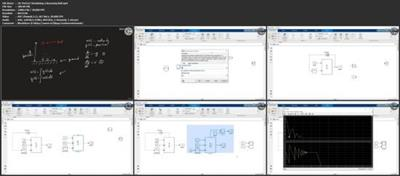MATLAB/Simulink Masterclass for Science and EngineeringGenre: eLearning | MP4 | Video: h264, 1280x720 | Audio: AAC, 48.0 KHz
Language: English | Size: 1.16 GB | Duration: 3h 46m
Learn how to use MATLAB and Simulink from scratch with several MATLAB code and Simulink model examples

What you'll learn
How to use MATLAB and Simulink for science and engineering applications
How to define variables and vectors in MATLAB
How to perform arithmetic and matrix operations in MATLAB
How to define trigonometric functions in MATLAB
How to make 2D and 3D Descriptions in MATLAB
How to program functions in MATLAB using for loops and if/else statements
How to model mechanical and electrical components in Simulink
How to run simulations of real-life processes and devices in Simulink

Description
This course is designed to allow you to learn how to use MATLAB and Simulink for Science and Engineering applications. The course is divided into the following sections:

1. Introduction to MATLAB/Simulink: in section 2 of the course, we will be downloading and installing the free MATLAB/Simulink trial to follow along with the course. We will also be discussing the MATLAB and Simulink environments and how they are used.

2. MATLAB for Science and Engineering: in section 3, we will be learning how to use MATLAB in a great amount of detail. We will begin by talking about how to execute simple commands, and continue working on more and more complex operations such as manipulating matrices and performing matrix operations, using trigonometric functions, and Descriptionting 2D and 3D Descriptions. We will also be discussing how to create programs and will be using For loops and If/Else statements. We will end the section by completing a few projects to make use of what we have learnt. The MATLAB code will also be provided so that you can follow along.

3. Simulink for Science and Engineering: in section 4 of the course, we will be learning how to use Simulink, a powerful modeling software commonly used in industry and academia. We will be learning about the library browser, which is where all the model components are located. We will then continue to build models, show how signals can be manipulated in Simulink, and run the simulations and the take measurements. We will also be learning how to use MATLAB functions inside of the Simulink environment. We will end the section by completing several projects. Note that the finished Simulink models will be provided so that you can follow along as well as use these models as a starting point for your projects.

As mentioned above, in each section, we will go over several projects to illustrate how we can use MATLAB/Simulink for different applications in science and engineering. The MATLAB code and Simulink models are also available for download so that you can follow along, as well as use these and modify them for your applications.

By learning how to use MATLAB/Simulink, you will be able to further your career in science and engineering.

Remember that Udemy offers a 30-day money-back guarantee. I am also always available for questions while you go through the course to ensure everything is clear.

See you in the course!

Who this course is for: